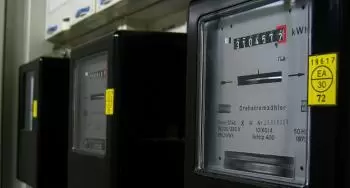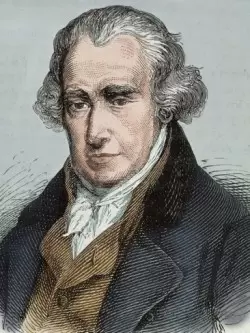# What Is the SI Unit of Power in Electricity? Watt DefinitionThe watt is the unit of power of the International System of Unit (SI), and its symbol is W. Its value indicates the amount of energy measured in joules that is converted, used, or dissipated in one second.

One watt is equivalent to 1 joule per second (1 J/s).

When working with very high powers, it is common to speak of kilowatts (kW), megawatts (MW), or gigawatts (GW).It is a derived unit of measurement named after the Scottish engineer, inventor, and instrument builder James Watt, for his contribution to the development of the steam engine.

The watt was adopted by the Second Congress of the British Association for the Advancement of Science in 1889. This power unit was defined in the International System of Units in 1948 at the 9th General Conference on Weights and Measures.

## The Formula for Electrical Power

In electricity, the watt is the unit of power of a system with a current intensity of one ampere under a voltage of one volt. It is the product of the voltage by the current flowing throw an electrical circuit.

P(t) = I(t)·U(t)

where:

• P(t) is the power as a function of time “t” expressed in watts.

• U(t) is the electrical voltage as a function of time “t” expressed in volts.

• I(t) is the electric current as a time “t” function expressed in amperes.

We can speak of average, effective, or instantaneous power depending on the nature of the current and voltage and their variation over time.

In an alternating current system, power is expressed in a particular way due to the periodic nature of the functions involved. We can establish different magnitudes such as real, reactive, complex, or apparent power.

## Difference Between an Electrical Watt and a Thermal Watt

Sometimes a difference is made between electrical watt and thermal watt to clarify whether we are talking about electrical or thermal power generated.

The term electric watt (symbolized We) corresponds to electrical power production. The term emphasizes that we only speak of electricity generation power regardless of the heat generated. Common multiple power units are the megawatt electric (MWe) and the gigawatt electric (GWe).

Thermal watt (symbolized Wt) is the unit of thermal power. With this concept, we want to highlight that we only discuss the heat generated. Its most common multiples are the thermal megawatt (MWt) and the thermal gigawatt (GWt).

This type of differentiation is often used in energy production, for example, to differentiate between the production of electricity and the thermal dissipation of a power plant.

## Difference Between Watt and Watt Hour

Watt is the unit of power, and a watt-hour is a unit of energy. A watt-hour is the rate at which energy flows when we exert a power of one watt for one hour.

For example, if a 10-watt light bulb is on for one hour, the power consumption will be ten watt-hours (Wh), equal to 3,600 joules (J).

The capacity or power of a power plant is measured in watts, but the electrical energy generated annually will be measured in kilowatt-hour (kWh).

## Other Power Units

There are more power units in the international measurement system than the watt (W). Some examples are:

• Units of power of the English system: horsepower (hp). Horsepower conversions are as follows:
1 HP = 550 ft lbf/s
1 HP = 745.7 W

• In the technical system of units, power can be expressed in kilograms per second (kgm/s). The conversion to watts is as follows:
1 kgm/s = 9.806215 W

• The power unit can also be expressed in kilocalories per hour (kcal/h). In this case, kilocalories can be converted to watts as follows:
1 kcal/h = 1000 cal/h = 1.1630556 W (watt).

• In the cegesimal system we have the erg per second, (erg/s). In this case, 1 erg/s = 1x10-7 W.

• Foot pounds: one foot-pound-force per second is equivalent to: 1.3558179483314 watts.

• Horsepower (CV): the conversion from horsepower to watts is as follows: 1 HP = 735.35375 W.

Author:

Published: May 13, 2015
Last review: September 13, 2022